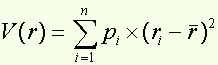Volatility calculation

Around the formula...

The risk carried by a security can be looked at in terms of the dispersion of its possible returns around an average return. Consequently, risk can be measured mathematically by the variance of its return, i.e. by the sum of the squares of the deviation of each return from expected outcome, weighted by the likelihood of each of the possible returns , or: